# Digital Test Chip for IBM 180nm 7RF Process

The purpose of this project is to design, submit for manufacturing and characterize a Digital Test Chip with Ring Oscillators in order to test drive the IBM 180nm 7RF CMOS process. It is important to know the limits of the process and having a confidence that specific crucial blocks that we want to use in more complex designs are doable on a given process.

That is why the first chip I developed for the IBM 180nm 7RF process was a test chip containing the following circuits:

• Ring Oscillators with fixed 1.8V Voltage Supply
• Ring Oscillators with variable Voltage Supply
• Voltage Level Shifters
• ESD Protection Cells
• Power Clamps

The design, simulation and experimental results of each block of the Digital Test Chip will be covered below.

## Ring Oscillator

The ring oscillator is a circuit composed of odd number of inverters, where output of the last inverter is connected to the input of the first inverter. In this configuration ring oscillator is oscillating with specific frequency which depends on the process parameters, supply voltage, the way how each inverter in a chain was sized and the propagation delay of each inverter.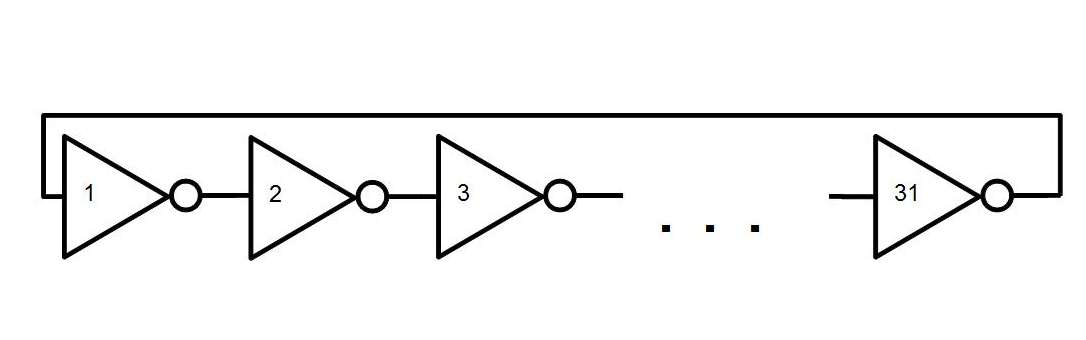Each inverter is composed of PMOS and NMOS transistors with minimum length for speed. The inverter configuration and sizing are shown below.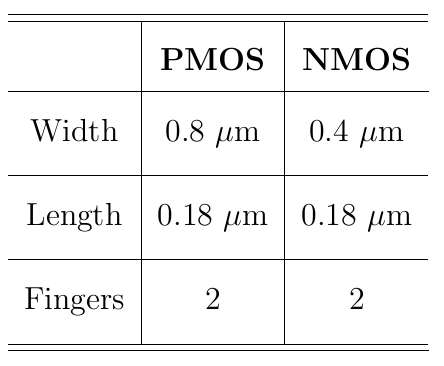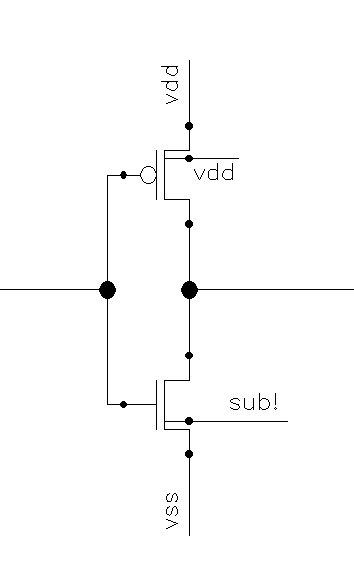The oscillation frequency of the ring oscillator depends heavily on the delay represented by each inverter in a chain. Using the method of logical effort, the delay can be calculated as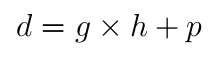Where d is the unitless delay, g is the logical effort, which is ratio of gate input capacitance to the unit inverter input capacitance. In our case the unit inverter is used, so the logical effort is 1. h is the electrical effort of inverter which is the ratio of output capacitance of the circuit to the input circuit capacitance. Since output and input unit capacitances of the unit inverter are close to 1 the electrical effort in this case has value of 1. p is the parasitic delay of an inverter, which is basically a delay represented by inverter when there is no load on the output, since I am using unit inverter our parasitic delay has a value of 1. Given the above values, the delay is computed as 2. I have N stages in the ring oscillator, signal propagates through the whole chain in circle to create oscillation, which is why I will be using 2N in the oscillation frequency formula.

The ring oscillator frequency can then be computed as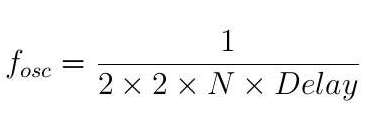The Delay in a formula above is the physical delay of each inverter measured during a simulation of inverter. Below is a figure showing that simulation.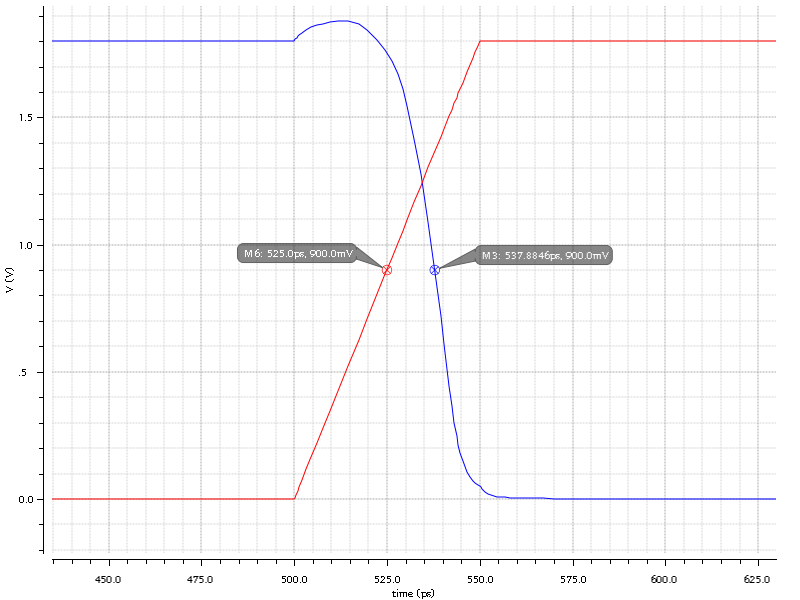Blue is output of inverter, Red is input signal to an inverter computing the distance between midpoint markers gives a value of 12.88 ps. The simulation was done under normal (tt) process corner in Cadence MMSIM simulator.

Knowing the physical delay - Delay, the number of stages - N and the unitless delay - d, I compute approximate oscillation frequency from the formula above as 626 MHz.

## Digital Buffer

The Digital Buffers are used for different reasons, sometimes to equalize the delays, isolate circuit from big external capacitive loads or driving the big capacitive loads. In our case I need a good digital buffer since the output of our the ring oscillator with unit sized inverters obviously won't be able to drive external load represented by PCB traces, PAD parasitic capacitance, Package parasitic capacitance, connectors, measurement equipment etc. In order to design a good buffer I first need to characterize better the unit inverter input and output capacitances.

### Unit Inverter Capacitance

I could compute the unit inverter gate input and output capacitances by calculating Cgd, Cgs, Cgdo and Cox knowing the approximate parameters of typical 180nm process, however; it won't be as precise as I want. That is why I will perform several types of simulations in order to figure that out.

First I want to approximate input capacitance, I am arranging the unit inverter testbench as shown below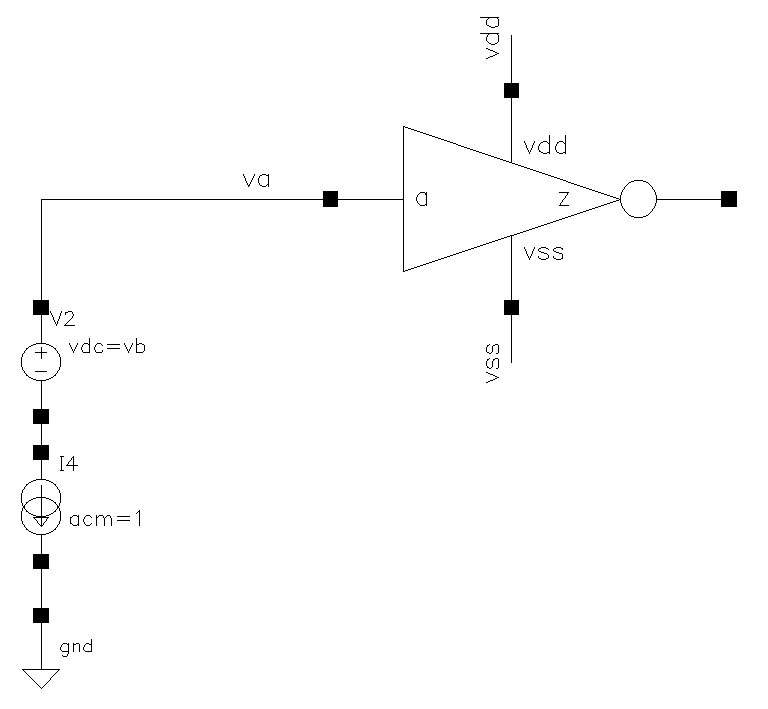The V2 source is used to provide different types of input bias voltage at the gate. I am using the following expression in ADE GXL to generate the plots of capacitance values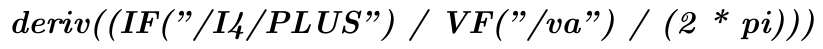Below is the plot obtained after AC simulation under normal corner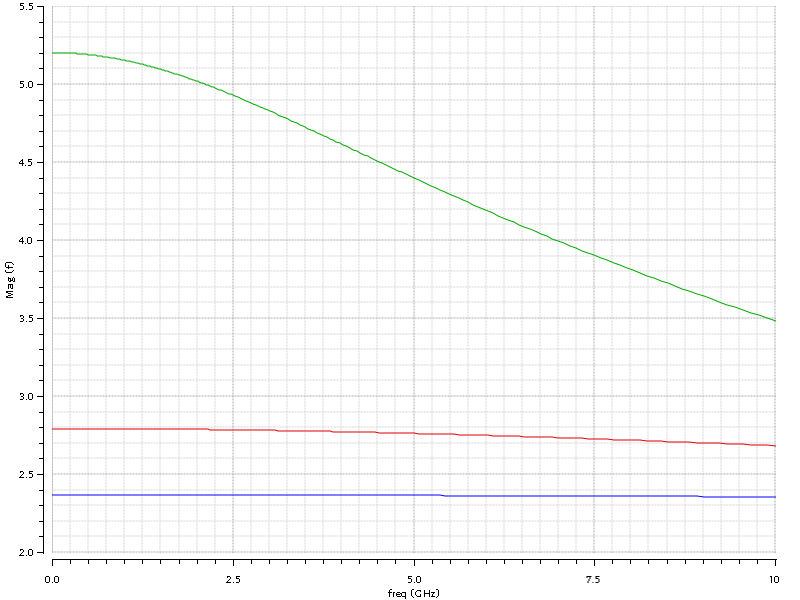Red: vb is at 0V, Green: vb is at 0.9V, Blue: vb is at 1.8V. The plot is shown for a wide range of frequencies. We can see that at input voltage of 0V and 1.8V it is pretty constant up to 10 GHz.

Next, the testbench was re-arranged as shown below.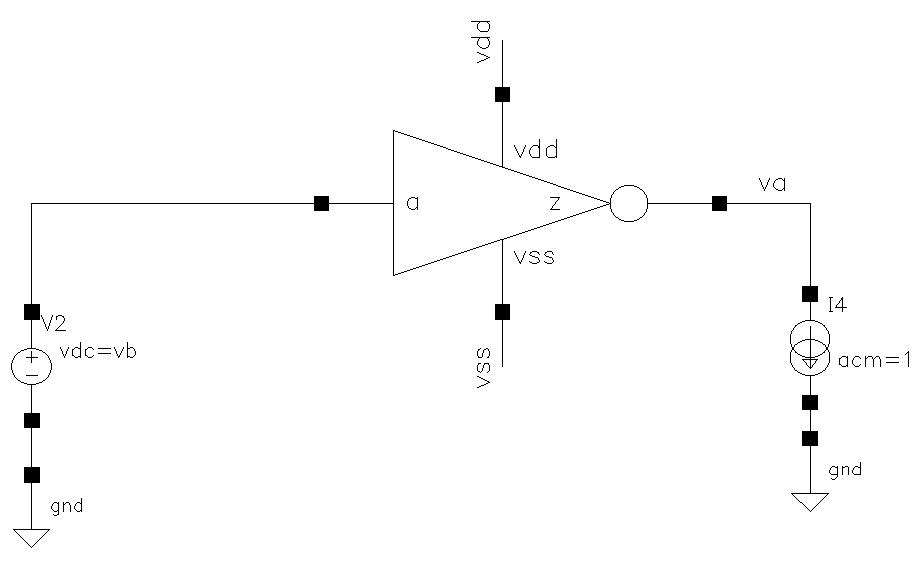The capacitance plot is shown below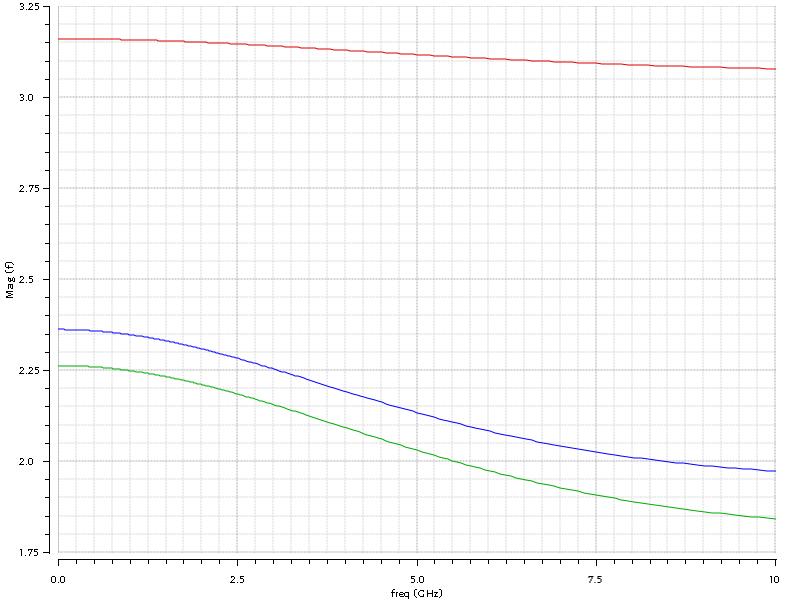Red: vb is at 0V, Green: vb is at 0.9V, Blue: vb is at 1.8V. If we take a ratio of output to input capacitance at bias voltages of 0V and 1.8V we can see that they are almost close to 1, a value which is supposed to be an electrical and logical effort of a unit inverter. At this stage I can also assume the unit capacitance C to be around 2.7 fF.

### Design and Optimization

Now it is time to design an optimal buffer. In order to do that, I select typical output capacitance seen by the buffer. I decide it to be around 5 pF, which is a typical capacitance present at the chip pin when it is connected to another chips via the PCB trace lines.

In order to optimize the buffer we need to first decide the number of stages, and before doing that the total path electrical, logical and branching efforts have to be calculated. The branching effort is the ratio of total capacitance on the path node to the capacitance of the path. In my case, there are no branches so the branching effort is 1.

The total logical, electrical and branching efforts of the path are simply products of the logical, electrical and branching efforts at each node and are designated as G, H and B respectively. The total path effort is F and is computed as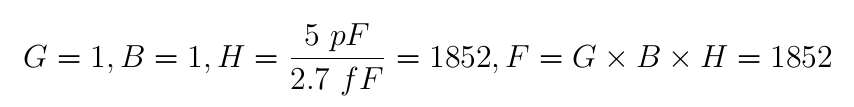2.7 fF is our unit inverter input capacitance, 5 pF is my target output capacitance seen by the digital buffer. Now I can start figuring out the number of stages for the buffer. It can be done using the following formula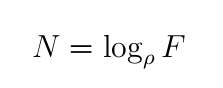where ρ is typically selected depending on the nature of circuit between 2.4 and 6. In my case, I choose it to be 4 for the buffer design. Computing number of stages given the above information gives N = 5.43, I ceil this value up to 6, so the number of stages I want for the buffer is 6.

The buffer will consist of 6 inverters. I have to size each of them appropriately. The first inverter will be a unit inverter, the one which was used in a ring oscillator. The others have to be sized based on the method of finding the best input gate capacitance given the output capcitance. The formulas for that method are shown belowThe g in each case will be equal to 1, f from formula above turns out to be 3.5, starting from the last stage input capacitance unitless size turns out to be 529.14, which means that the stage #6 of the buffer must be an inverter which is 529.14 times stronger than unit inverter. Now using the value of 529.14 as output unitless capacitance we calculate the input unitless capacitance of the inverter #5, and so on up to the first inverter, which must turn out to be a value close to 1. The table with all the computed values is shown below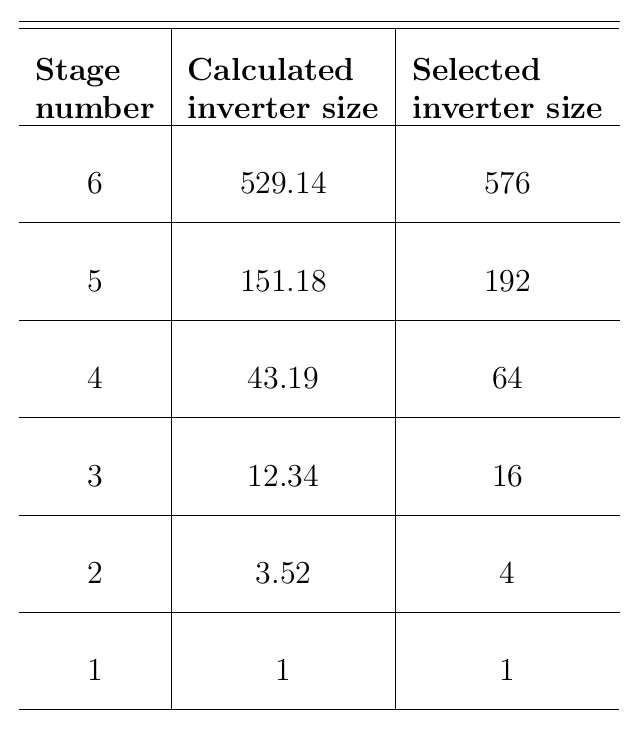After I calculated sizes, I ran some simulations and saw that waveform shapes are not sharp enough, I decided to further tweak the sizing of each stage. The table above shows both the calculated inverter sizes for digital buffer and also the size which I finally decided to choose.

Below is a schematic of the digital buffer with 6 inverter stages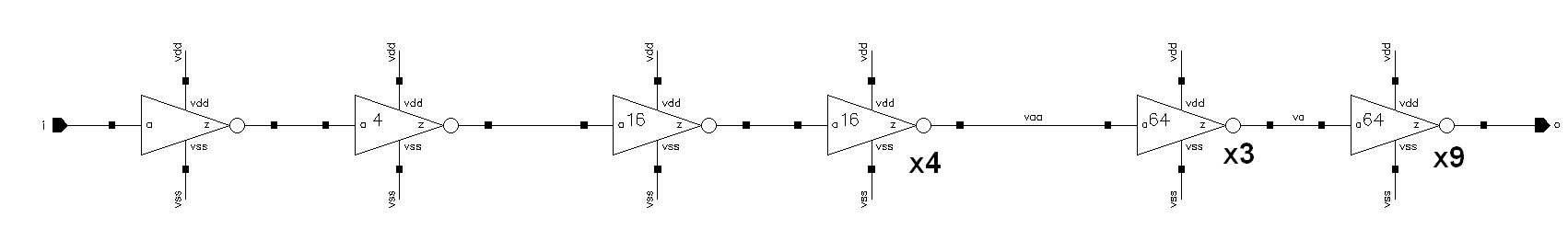You can notice that I used mainly buffers with size of 1,4,16 and 64. The last stage for example was implemented as 9 parallel inverters, where each inverter is 64 times stronger than the unit inverter.

## Voltage Level Shifter

The Voltage Level Shifter converts signals from one voltage level to another. In my case where I have a ring oscillators with variable power supply and output signals of lower voltage levels I need to convert those signals to a proper 1.8V voltage level before feeding them to a buffer. So I need to have a voltage level converter. The schematics of that converter is shown below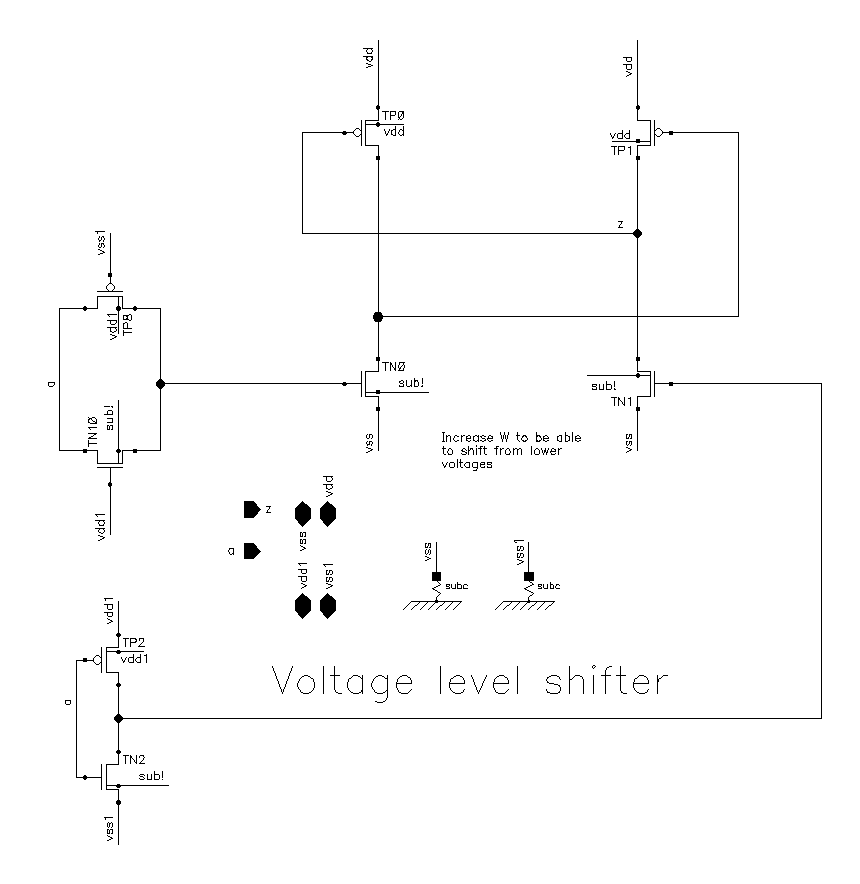The vdd is at 1.8V, the vdd1 is a voltage from external pin of the chip powering the ring oscillators with variable voltage supply. The ground rails vss and vss1 are connected to each other and are at 0V. Enabled transmission gates made up with TP8 and TN10 are used to equalize the delay represented by the inverter.

When the signal a is LOW TN0 is OFF and TN1 is ON forcing node z to LOW, that turns ON TP0 . As a result the gate of TP1 is HIGH turning it OFF.

When the signal a is HIGH, TN1 is OFF and TN0 is ON forcing the gate voltage of TP1 to LOW and turning it ON. That forces node z to HIGH and keeps TP0 OFF.

It is not the best voltage shifter design, it also consumes more power since it is always ON. For a low power applications the dynamical or clocked voltage level shifters are used. But for the current design this solution will do it.

Transistor sizing for the voltage level shifter is shown below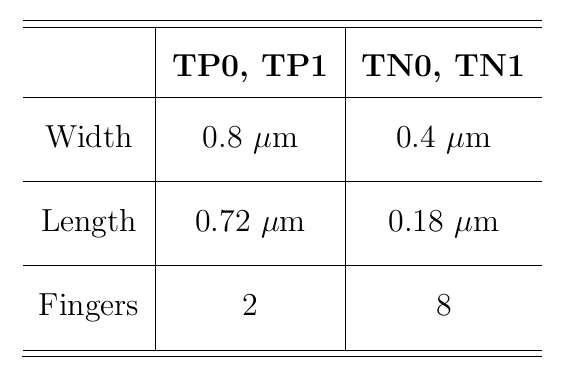## ESD Protection

It is mandatory to consider proper ESD protection for almost most of the chips. Electrostatic Discharge (ESD) is a high current pulse event which occurs when a chip comes in cotnact with the environment. The pins of the chip might get in cotact with the human body, different parts of the machinery during handling at the foundry or any other object carrying an electric current. I was refering to the ESD reference guide and design kit of IBM when integrating an ESD protection in my chip.

At least the HBM (Human Body Model) and MM (Machine Model) type ESD protection mechanisms are required to be used with a pads on the chip. Below is a block diagram picture of the ESD protection method used in this design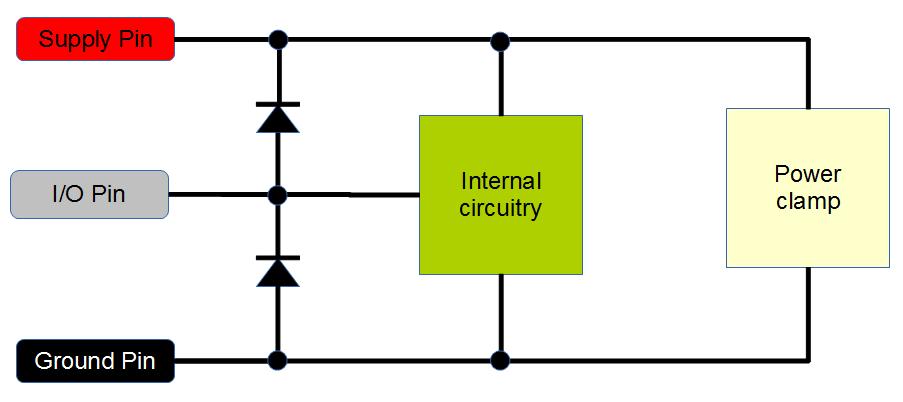There are two main ESD strategies, self protecting and non-self protecting. The self protecting strategy assumes that ESD current flows through the I/O circuit and the device within the I/O circuit can handle that current. A non-self protecting strategy assumes that ESD current is not flowing through the I/O circuit and an appropriate ESD device is added before the I/O circuit and does not let the current flow through it.

Our design utilizes the non-self protecting strategy by placing the ESD protection device at the input and output pads of the chip. The I/O pad voltage will not exceed the supply voltage during normal operation and the main supply will be powered before any signal is applied to a pad. The typical protection mechanism is shown above in the picture.

When a positive pulse occurs with respect to the supply pin, current travels through the top diode and exits the supply pin. When a positive pulse occurs with respect to the ground pin, current again travels through the top diode all the way to the ground pin through the voltage clamp.

Similarly, during a negative pulse with respect to supply pin current flows through the supply pin all the way to the I/O pin through the power clamp and bottom diode. During a negative pulse with respect to the ground pin, the current flows from the ground pin to the I/O pin through the bottom diode.

This way the excess current during the ESD events does not flow through the internal circuitry, thereby damaging it. The power clamp is a special circuit designed in such a way that it starts conducting only during an ESD event.

### The ESD Cell

The ESD cell is basically a series connected diodes at the input. Stacked diodes allows to handle voltages exceeding the power supply voltage. Below is a schematic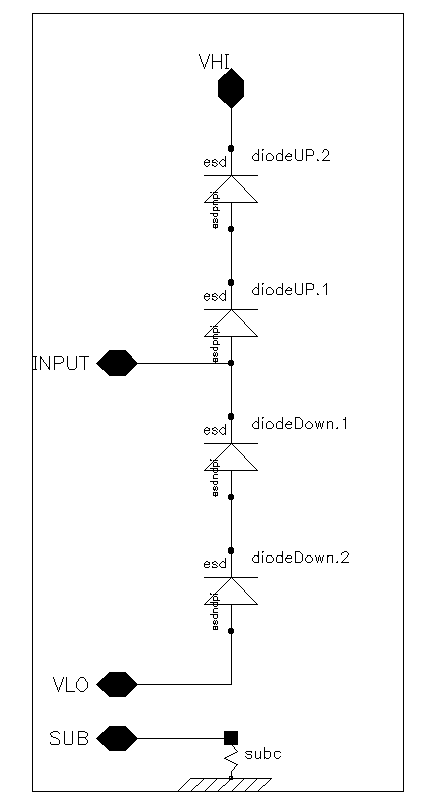### The Power Clamp

The power clamp consists of an RC network, inverter chain and large-sized NFET transistor. During an ESD transient event, the RC circuit detects the high current pulse and turns on the inverter chain. The last inverter turns on the big NFET transistor which handles the ESD current. The power clamp was con gured for a standard time constant of 0.96 s within the design kit. The time constant controls the power clamp on-time during the ESD event. Configured and automatically generated schematics are shown below in the picture. The power clamp is placed at every supply and ground pad of the chip.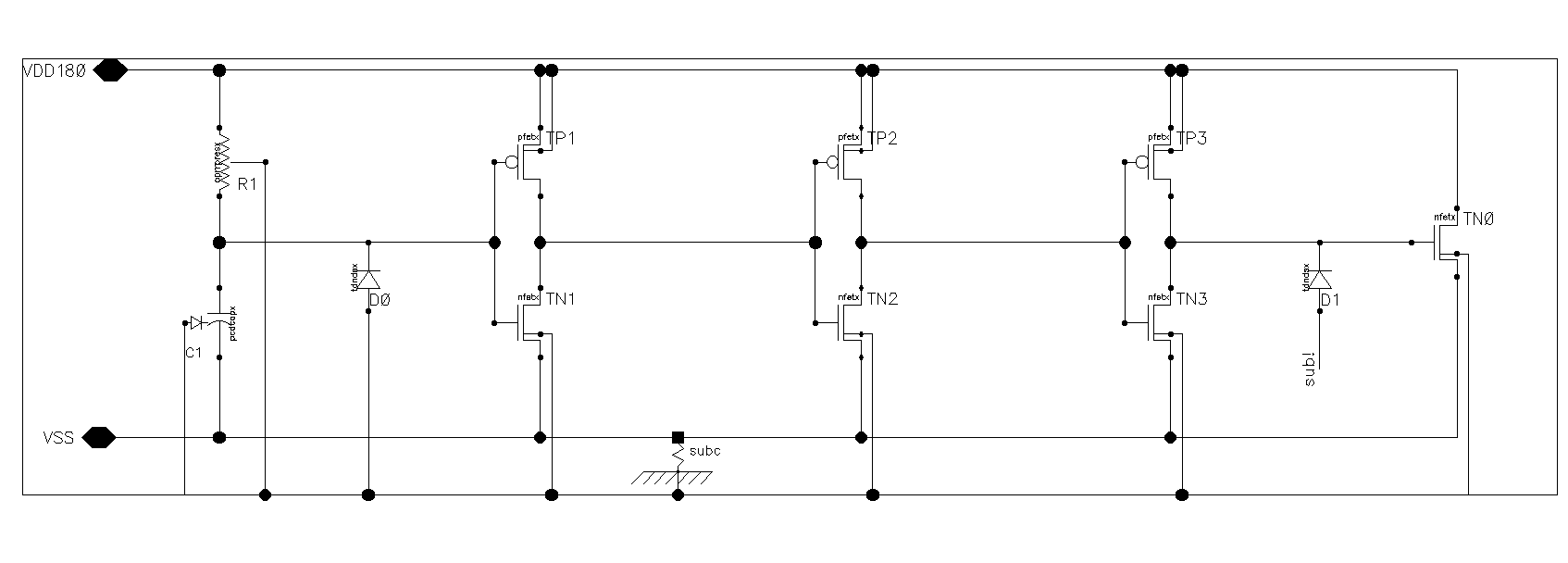## Simulation

Now it is time to show the simulation of each block and look at the results.

### Simulation of Ring Oscillator with fixed 1.8V Supply

The 31-stage Ring Oscillator was connected to 1.8V voltage source. The normal corner was selected for simulation. The frequency of simulation was observed to be 750.5 MHz compared to the 626 Mhz computed frequency using the result of simulation of inverter delay. The transient plot is shown below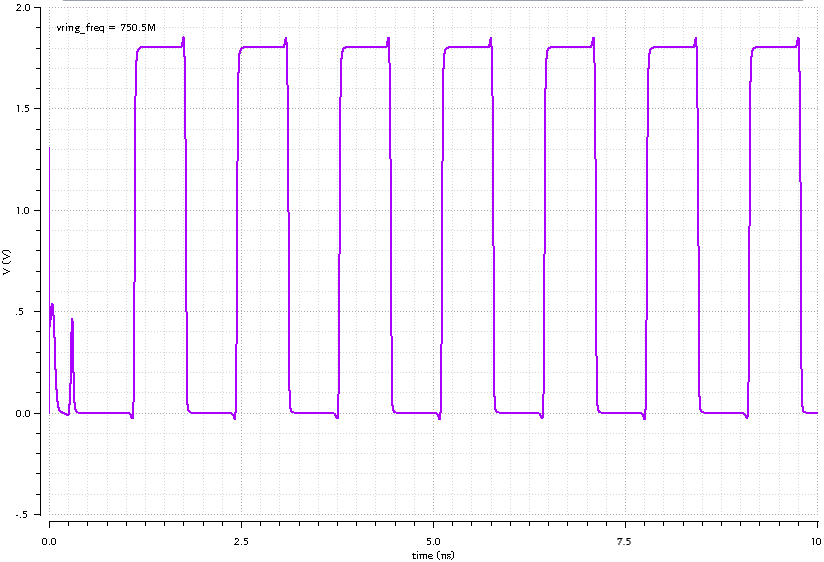### Simulation of Ring Oscillator with Variable Voltage Supply

In order to simulate the ring oscillator with variable voltage supply, I had to re-arrange the testbench as shown below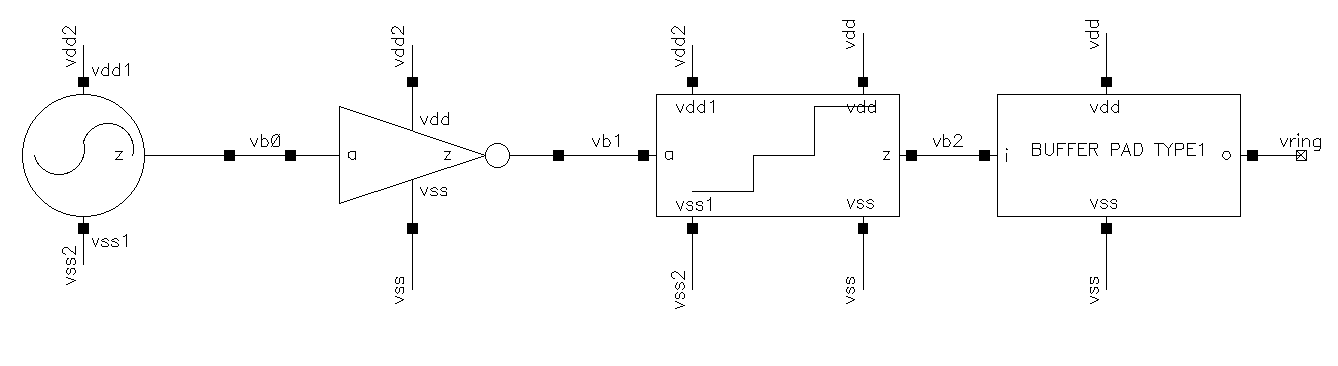The schematics of testbench starts with ring oscillator supplied with variable voltage source, the inverter which provides isolation of the output of ring oscillator from capacitance at the voltage level shifter, the voltage level shifter and the digital buffer. All the blocks have been designed and discussed above. The simulation transient waveform is shown below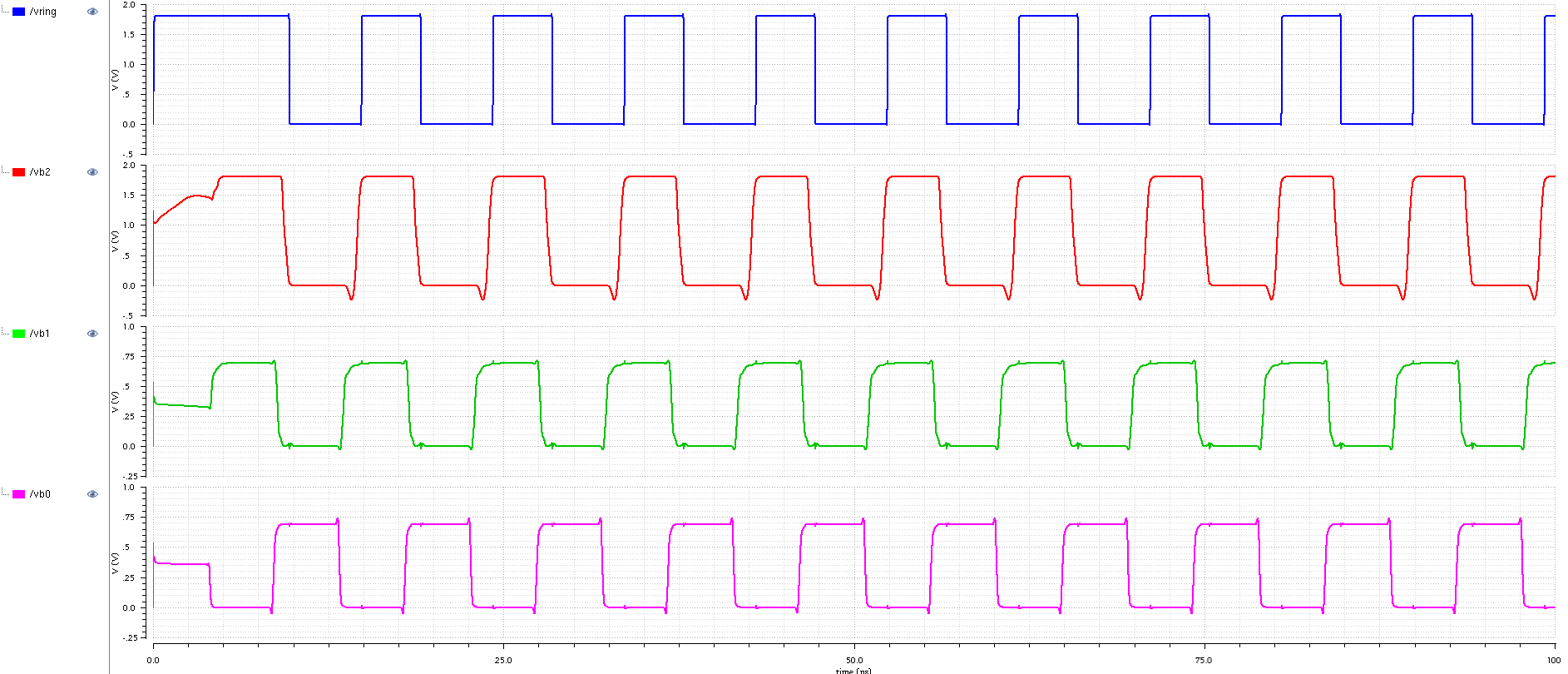The voltage of around 0.69V was provided to the ring oscillator, the output signal coming from it designated as vb0 has 0.69V level as well. Then it is being inverted as shown on vb1 waveform, after that its level is shifted to 1.8V as shown on vb2 waveform. Finally, it is passing a digital buffer which removed the undershoot, improved the rise/fall times and incereased strength of the signal. You can click on the simulation waveform picture to enlarge it in new window.

Initially I planned to use my HP 54510A scope with 250 MHz bandwidth and 1 GSPS sampling rate. So I performed the simulation of the variable ring oscillator in which the supply voltage was varied so that maximum frequency of 250 MHz is obtained with slowest corner. The simulation plot is shown below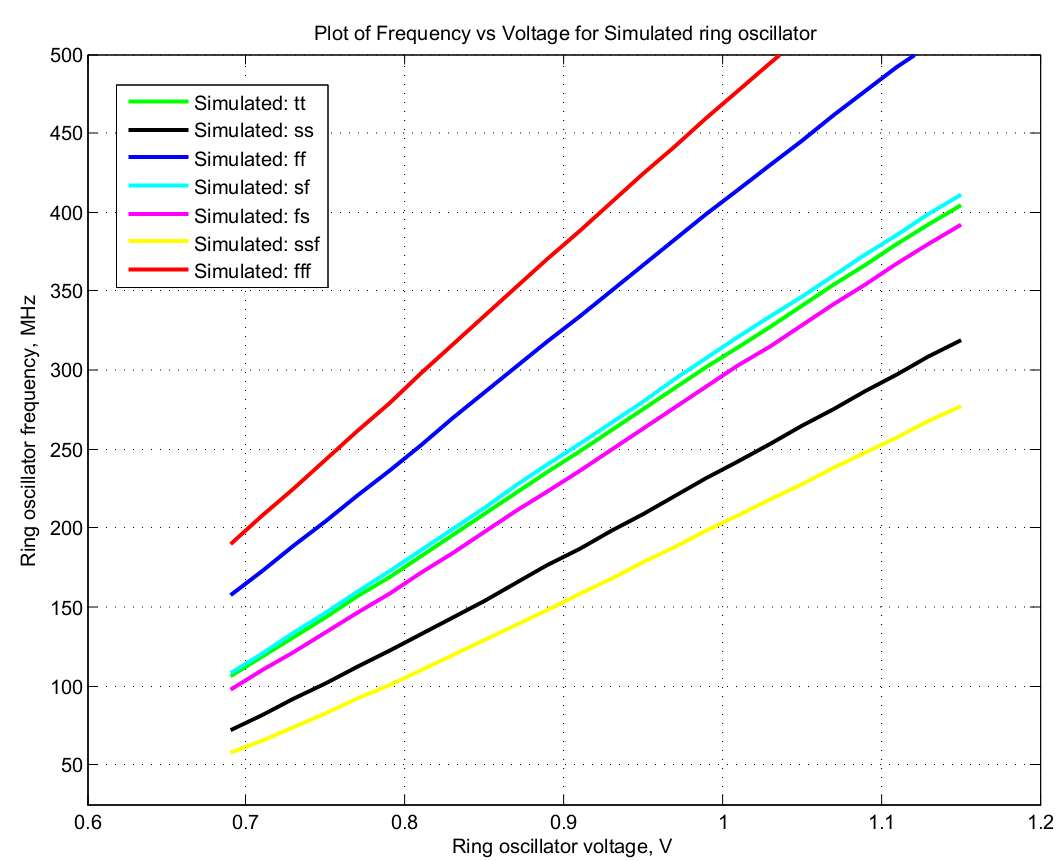The fff is the fastest corner, and ssf is the slowest corner.

### Simulation of Digital Buffer

The following testbench was constructed for the simulation of digital buffer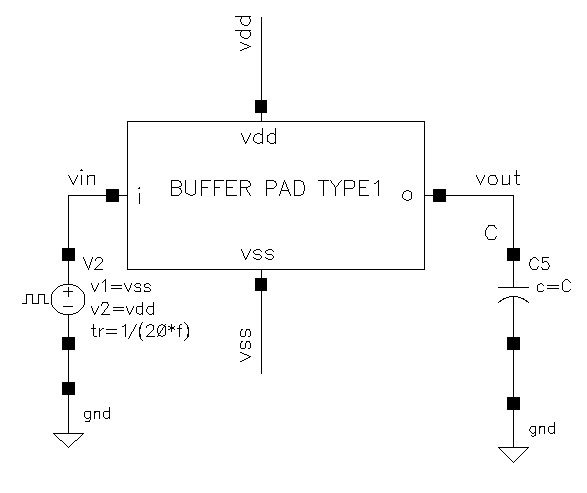The output capacitance was varied between 1 pF and 30 pF in increments of 1 pF. The plot below shows the input signal, and the output signals corresponding to different output capacitances. The higher the output capacitance, the less squared the shape of the output signal is, and more delay can be observed using the mid-point rule.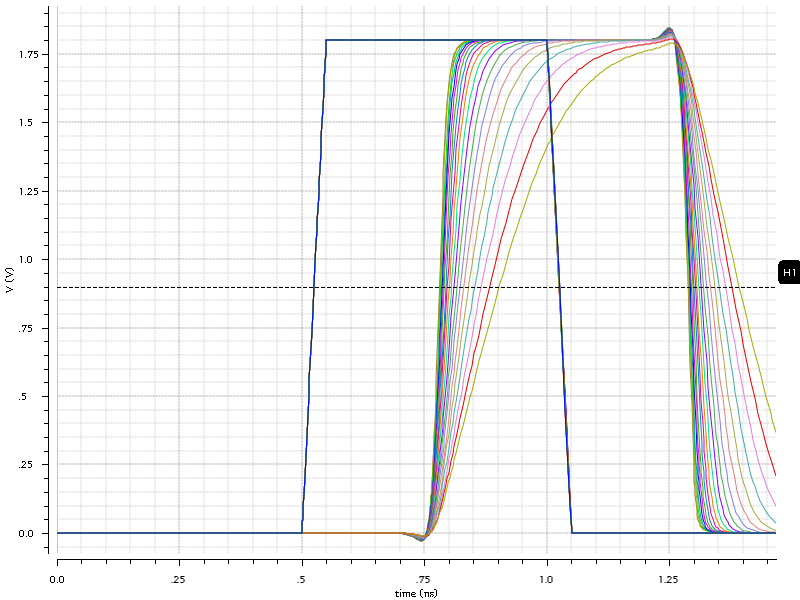The next plot shows the measured buffer delay based on the output capacitance variation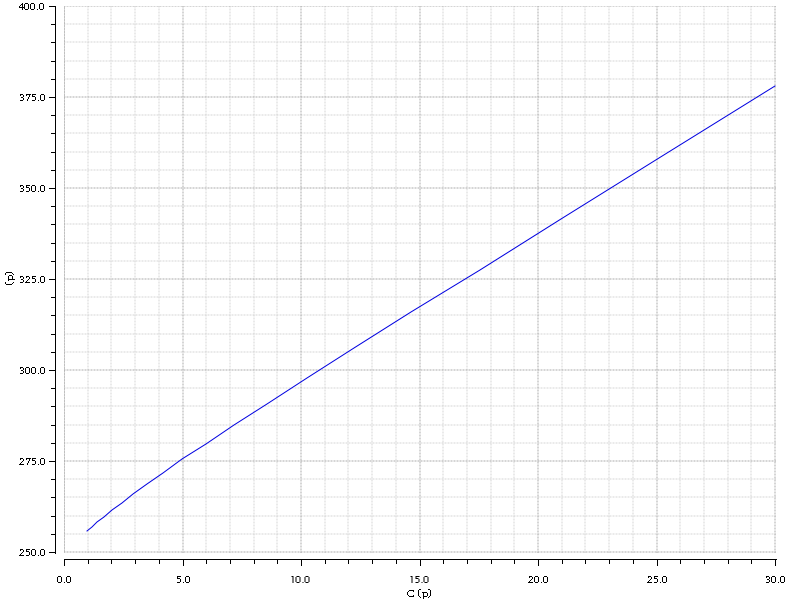So we can see that at around 30 pF output capacitance, the delay will be around 375 ps, not that bad for such a huge capacitance.

Next the buffer was simulated with the added ESD cell and PAD parasitics at the output, rough parasitic model of package and ideal transmission line with 50 Ohm impedance. The testbench setup is shown belowAnd below is the waveforms of the simulation at 250 MHz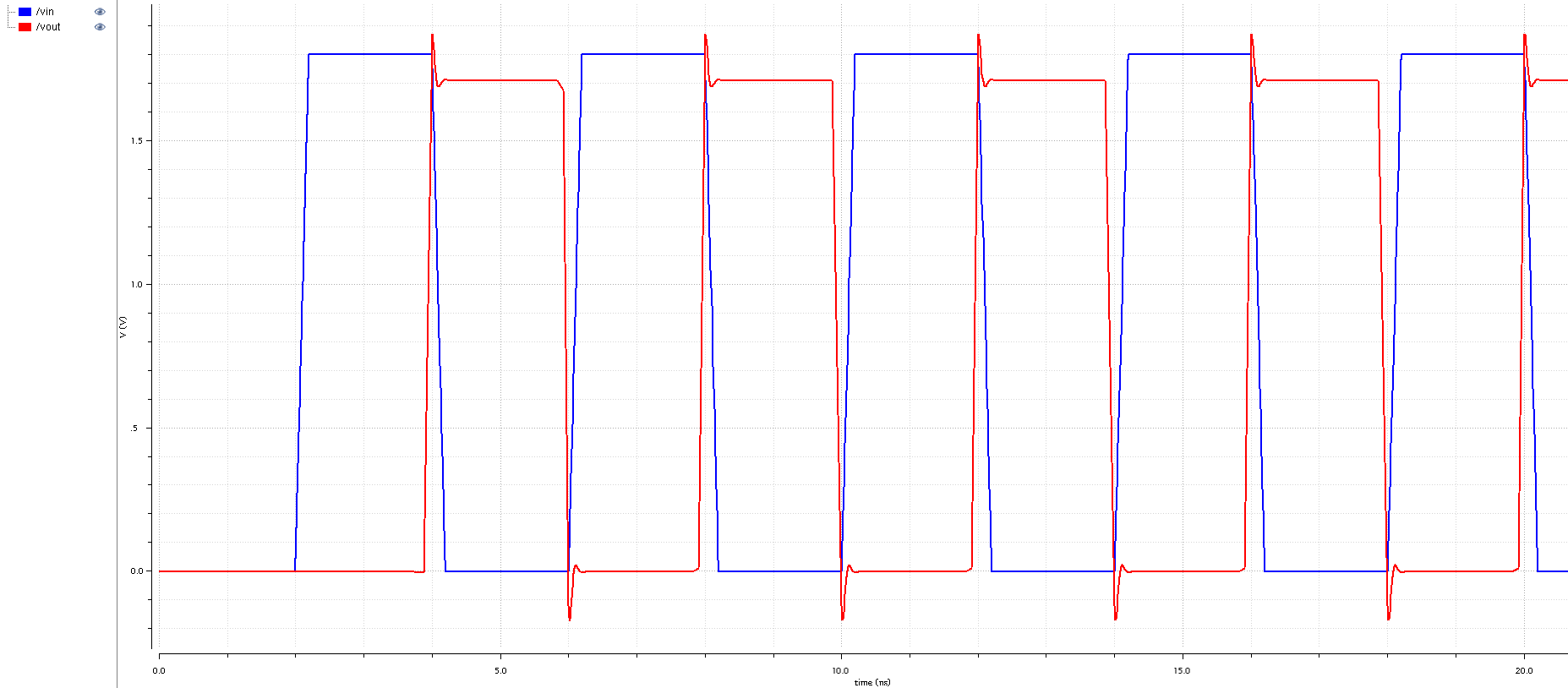And below is the waveforms of the simulation at 1 GHzAnd below is the waveforms of the simulation at 2 GHz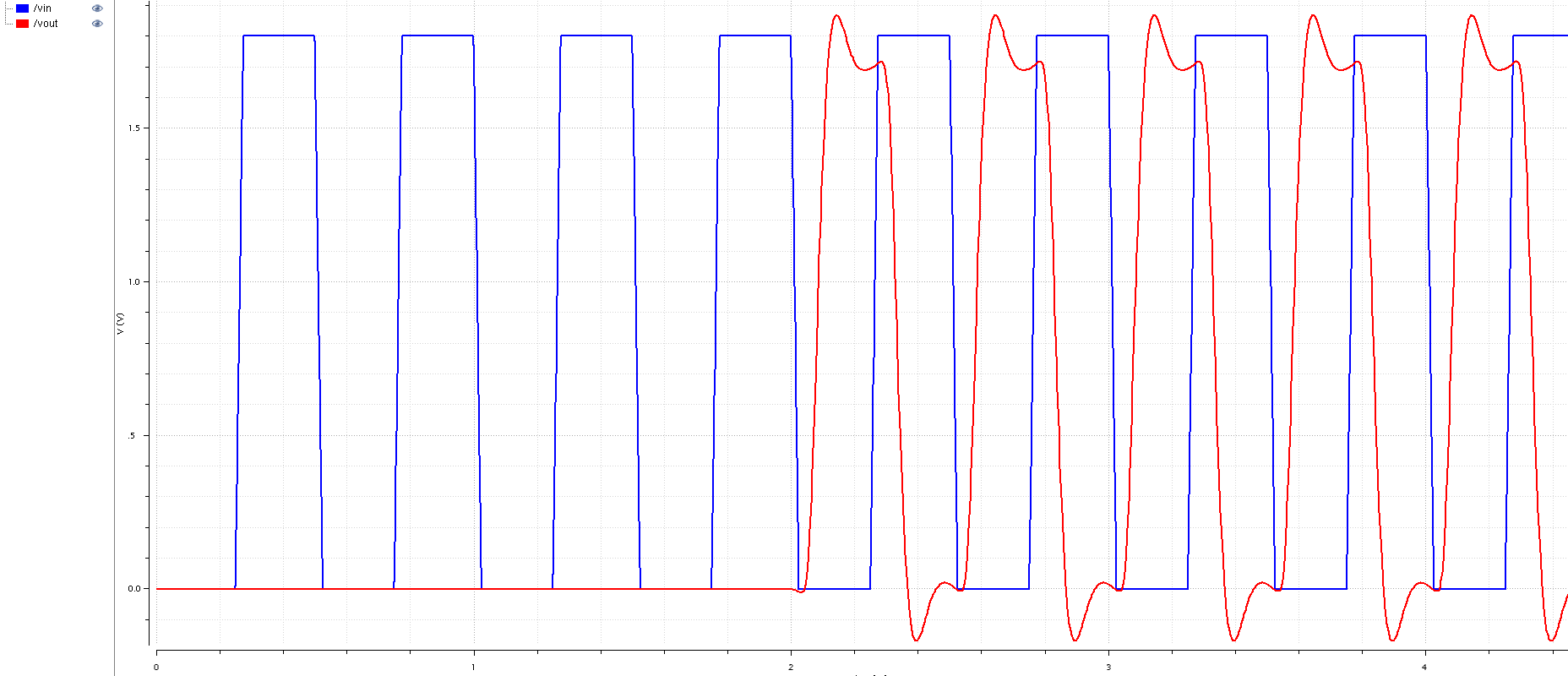And below is the waveforms of the simulation at 3 GHz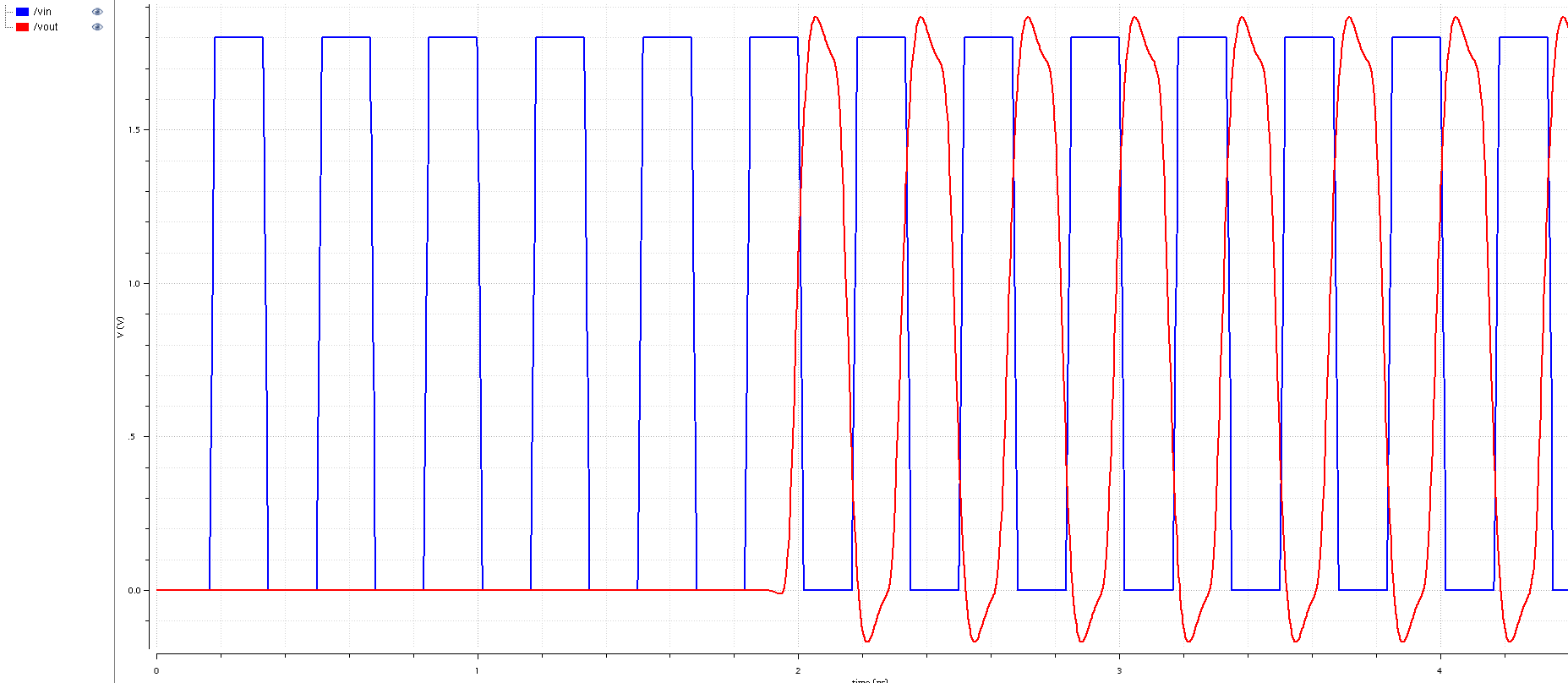It is important to have a nice, convenient and properly designed PAD Frame for the chips. Depending on the design of course they can be different. The total area of the chip I decided to use is 1.5mm x 1.5mm. For most of my tests designs under this process around 1mm x 1mm area is enough for my designs. The rest of the chip space is used by PAD frame, power supply rings, ESD cells, Power Clamps, Buffers, etc. As well as some hot looking logos. It's really important to have an awesome logos or the chips don't seem to be legit.

Below is a floorplan of the PAD frame I used, the actual design have of course rounded corners and several other details. I cannot show the layout snapshot due to the NDA nature of the IBM CMRF7SF Kit.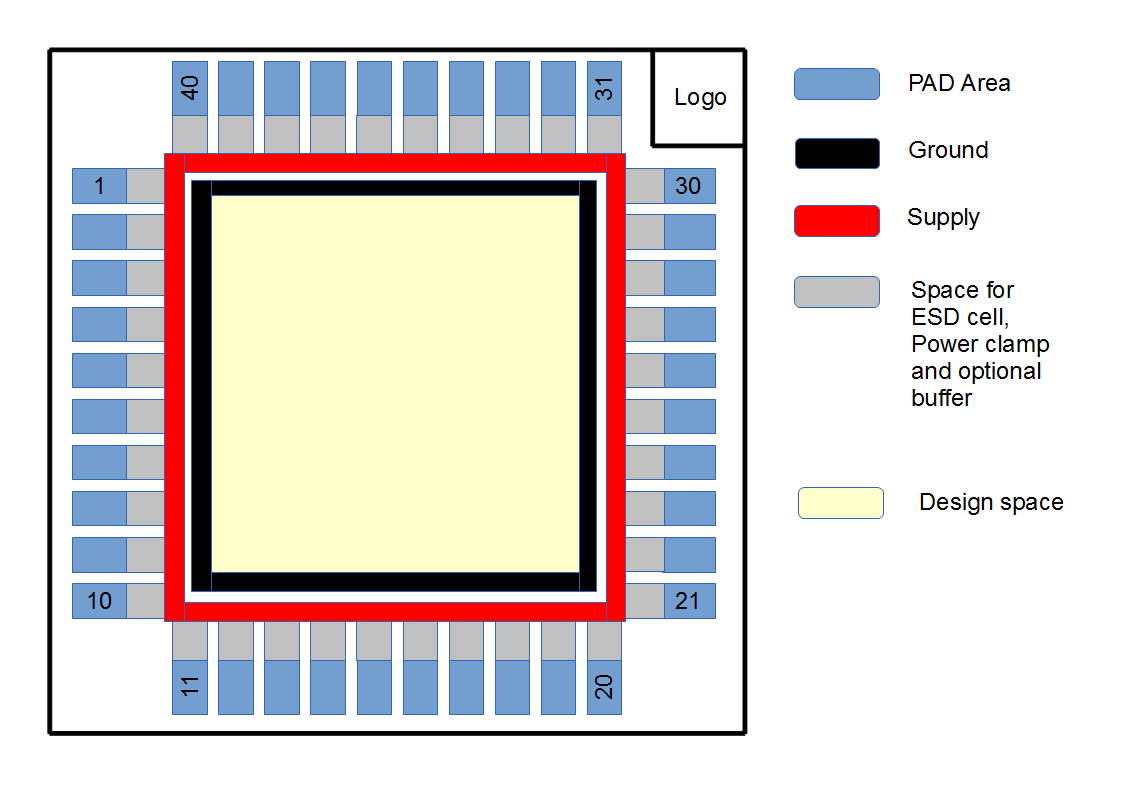## Test PCB

The schematics of the test PCB are below. I have total of 6 Ring Oscillators, 3 of them have fixed voltage supply, another 3 variable voltage supply.

### Test PCB Schematics

First page is primary DUT and its I/O signals.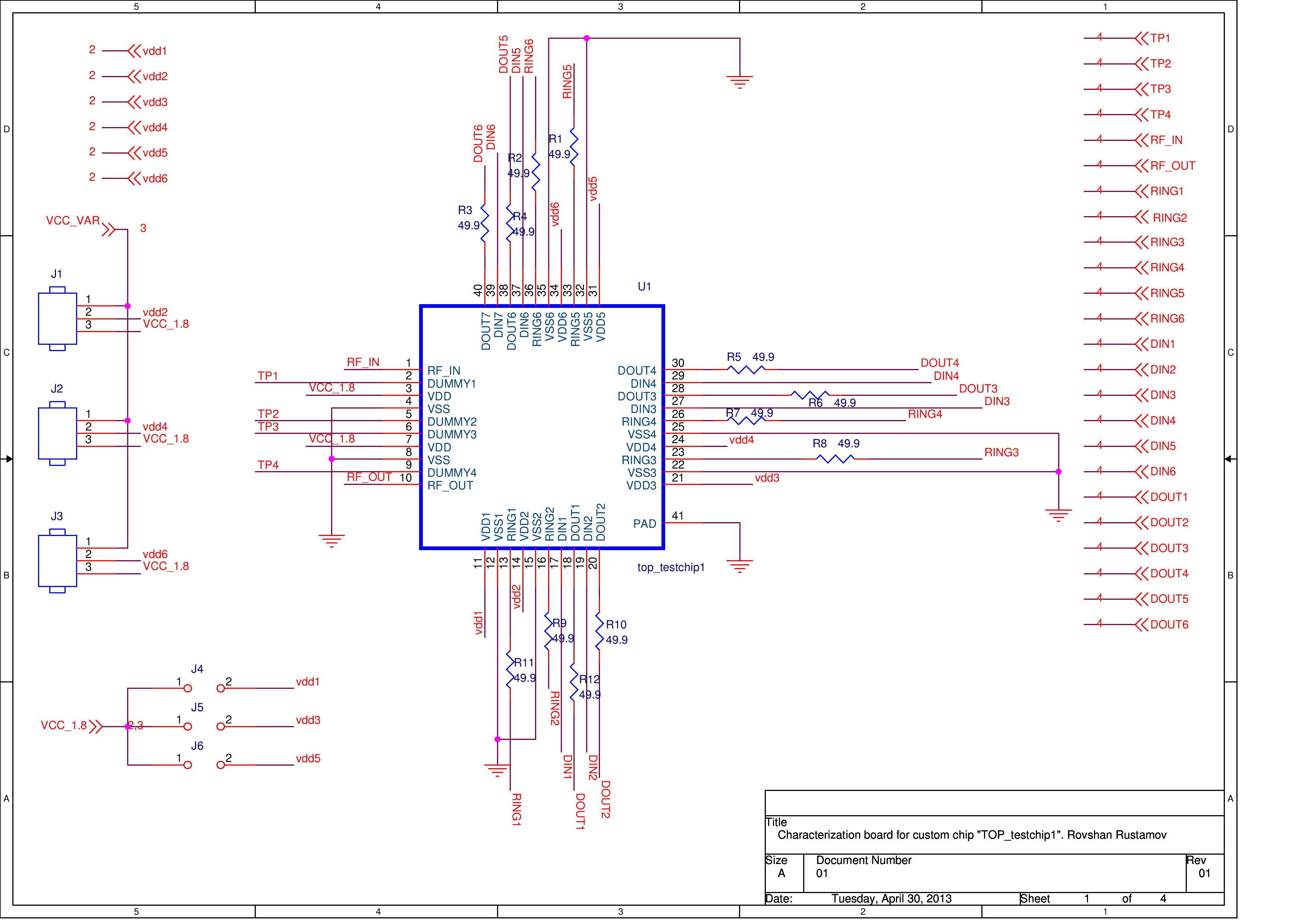This is the array of decoupling capacitors for each ring oscillator and for main power supply.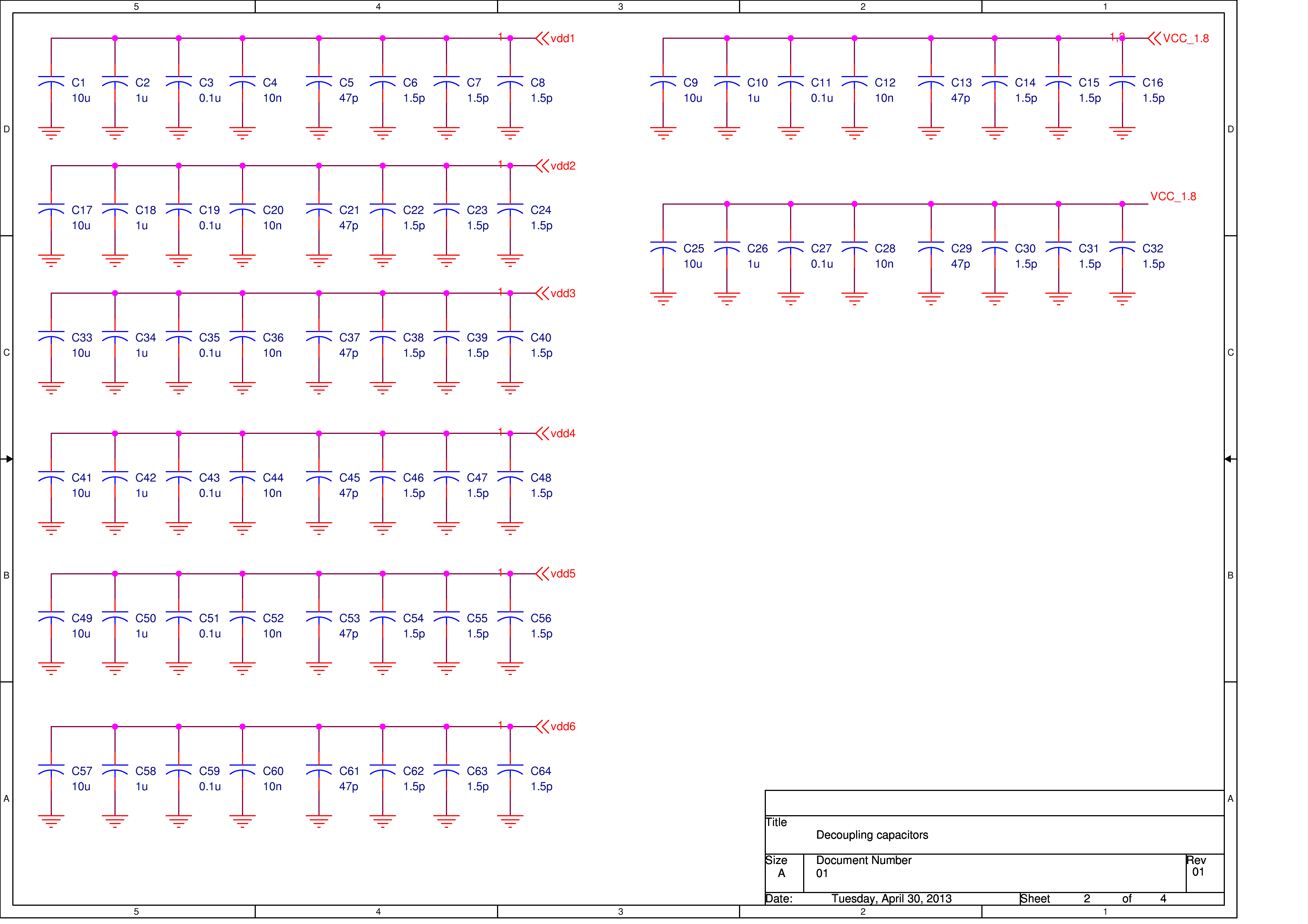The next picture shows on-board power supply with Linear Technology LDOs (My favourite LDOs!)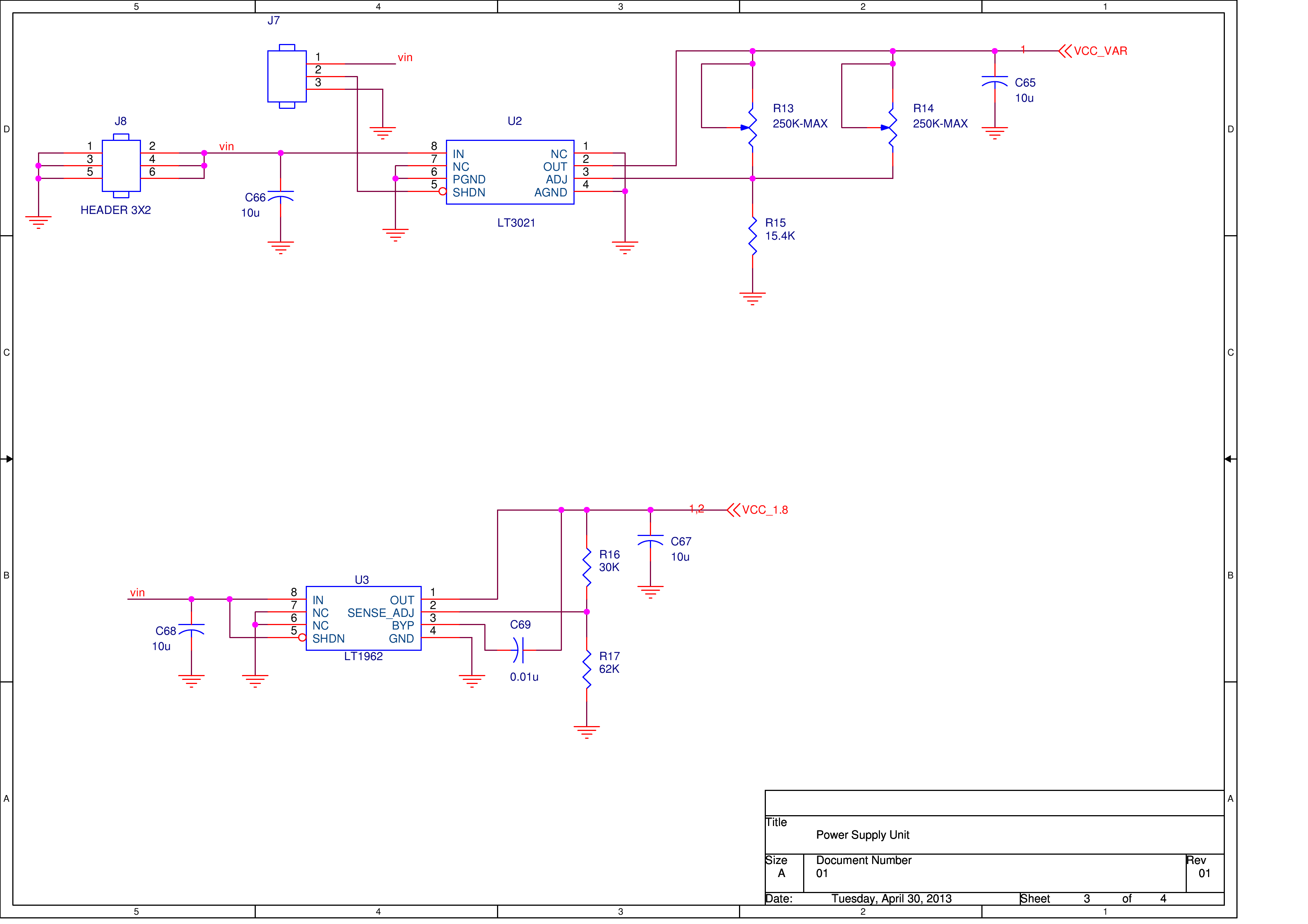SMA and other type of connectors.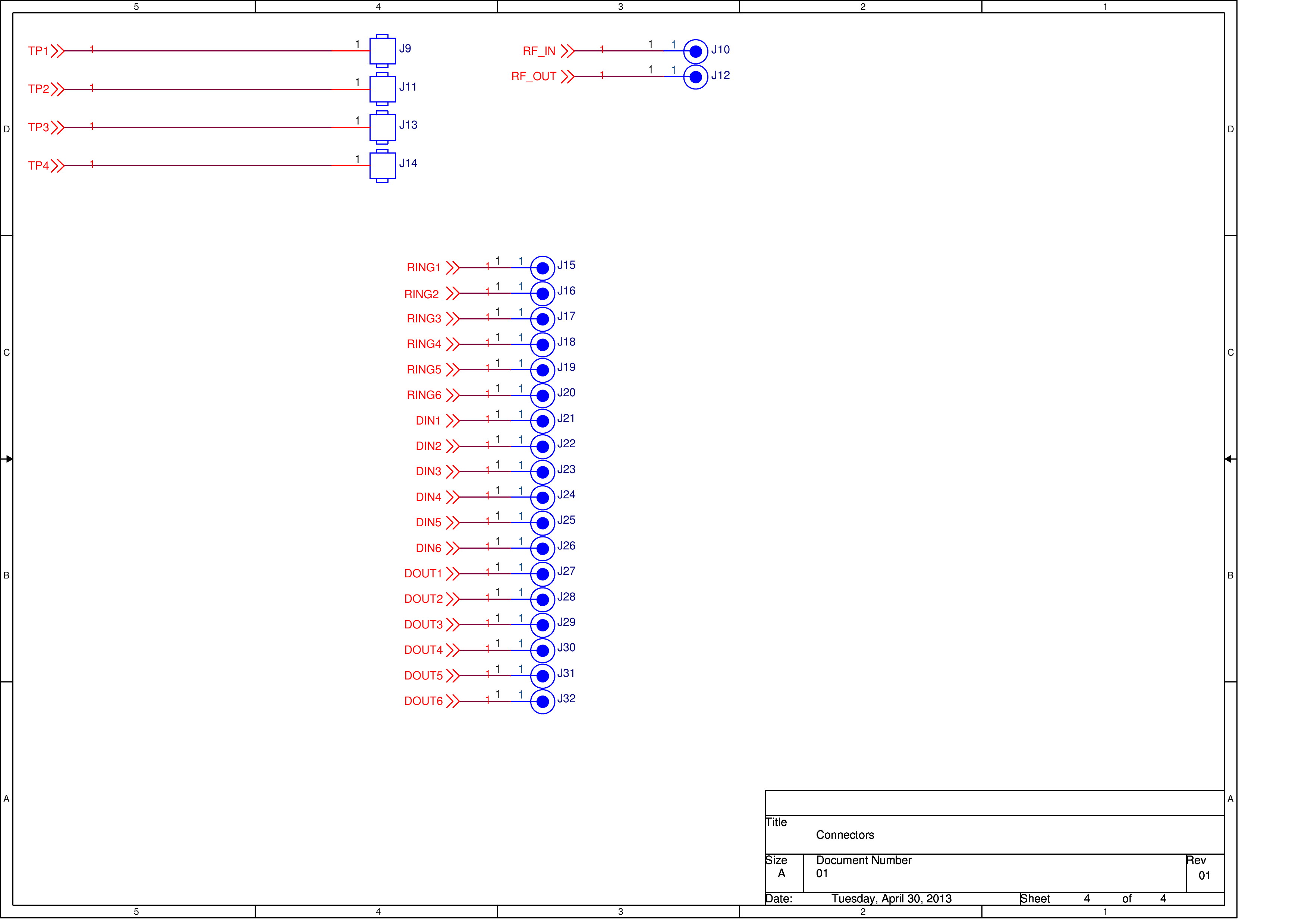### Test PCB Layout

Awesome PCB for Awesome chip, right? So, since I was planning to test the chip at its full working frequency I had to design a proper PCB. I decided to use standard FR408 process with 4 layers and dielectric constant 3.66. The rules were: 5 mil trace/space, and 10 mil minimum drill diameter. The stackup is shown below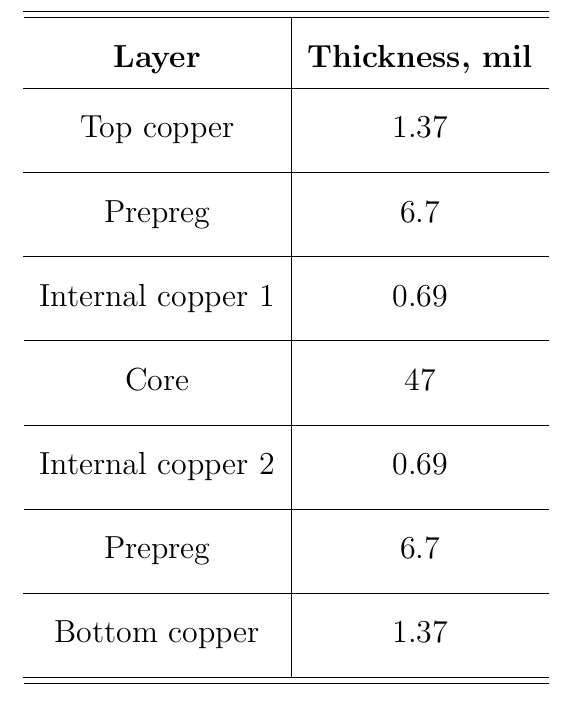### Gerber Snapshots

Below are the snapshots of the Gerber files. Top layer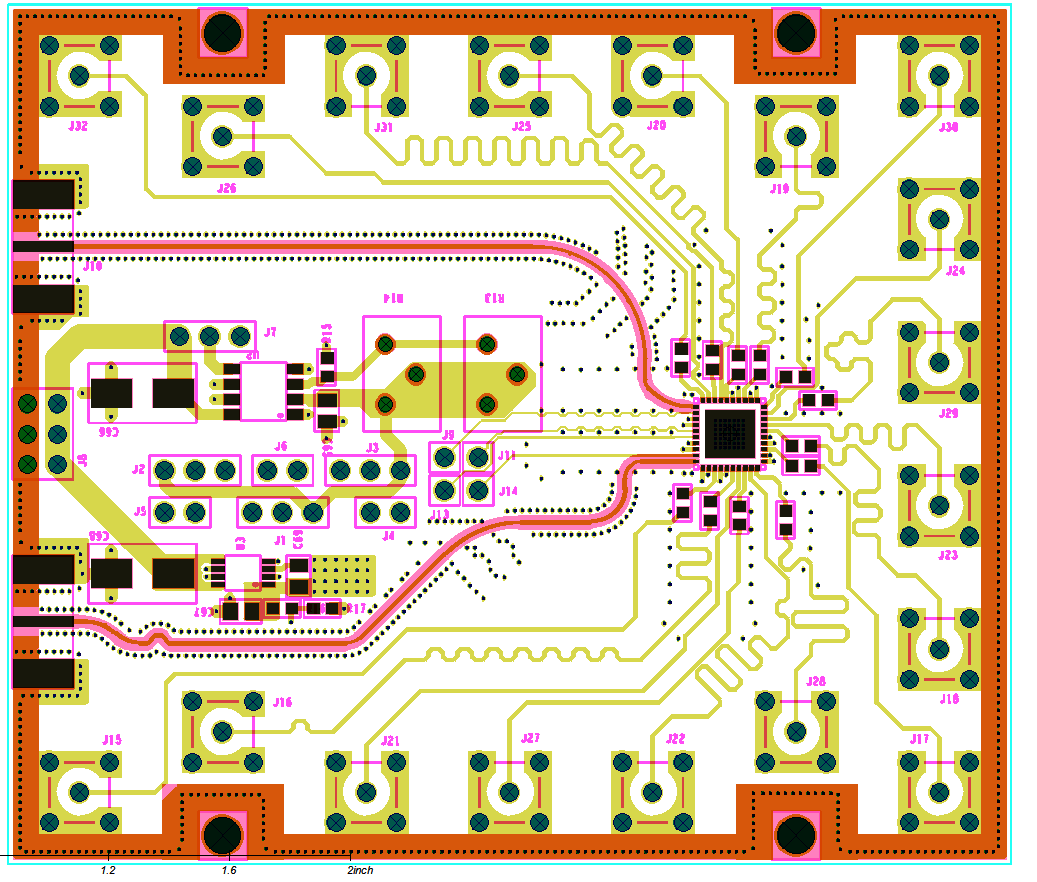Internal Layer 1, Ground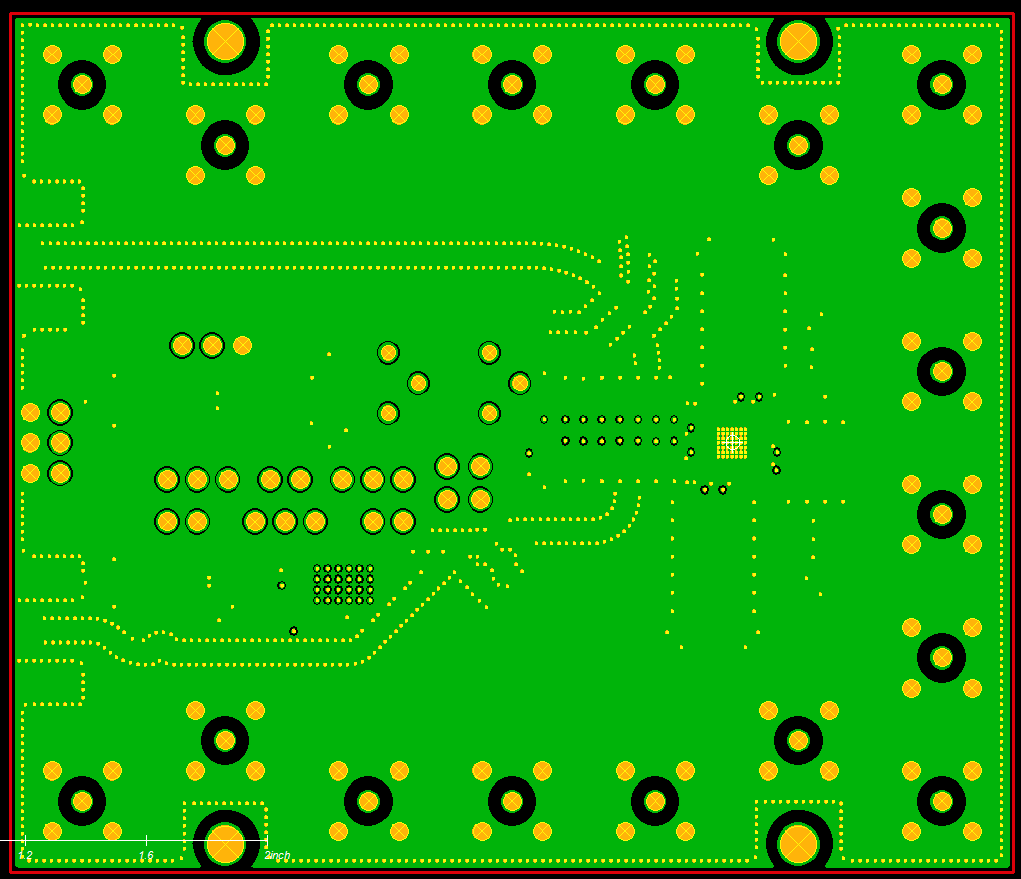Internal Layer 2, Power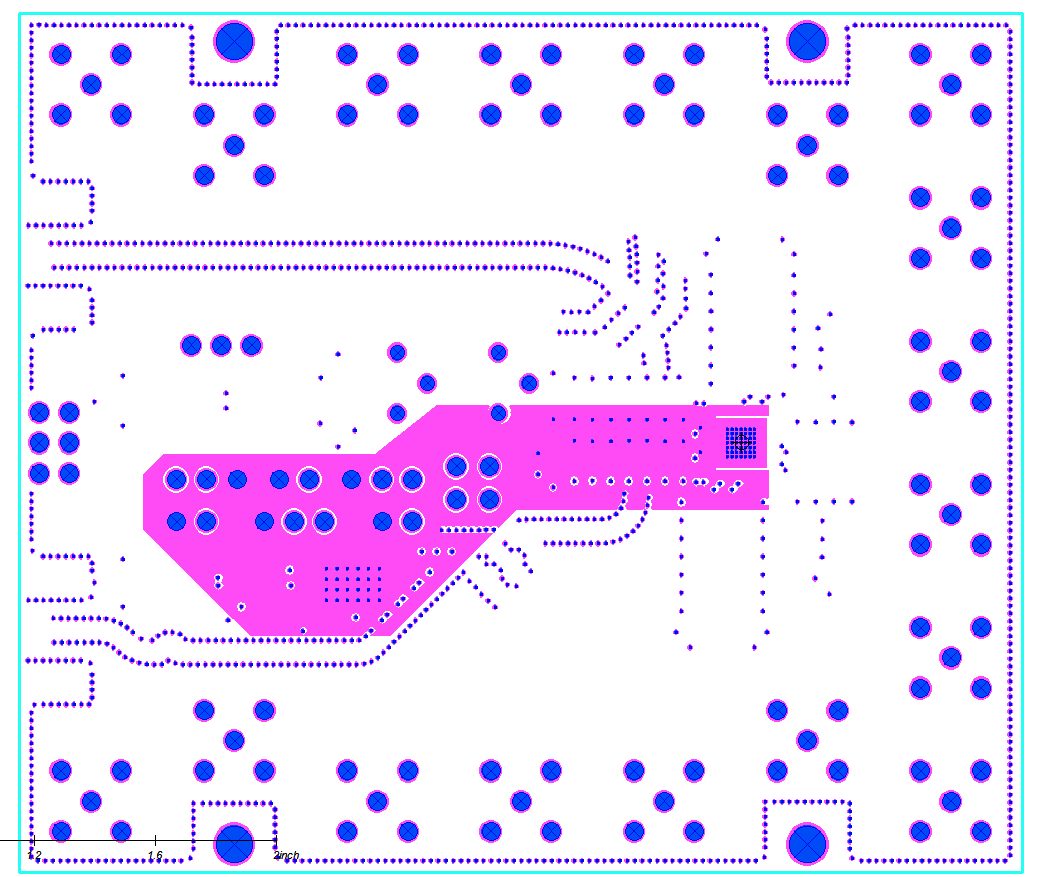Bottom Layer, here I routed variable supply Ring Oscillator's power lines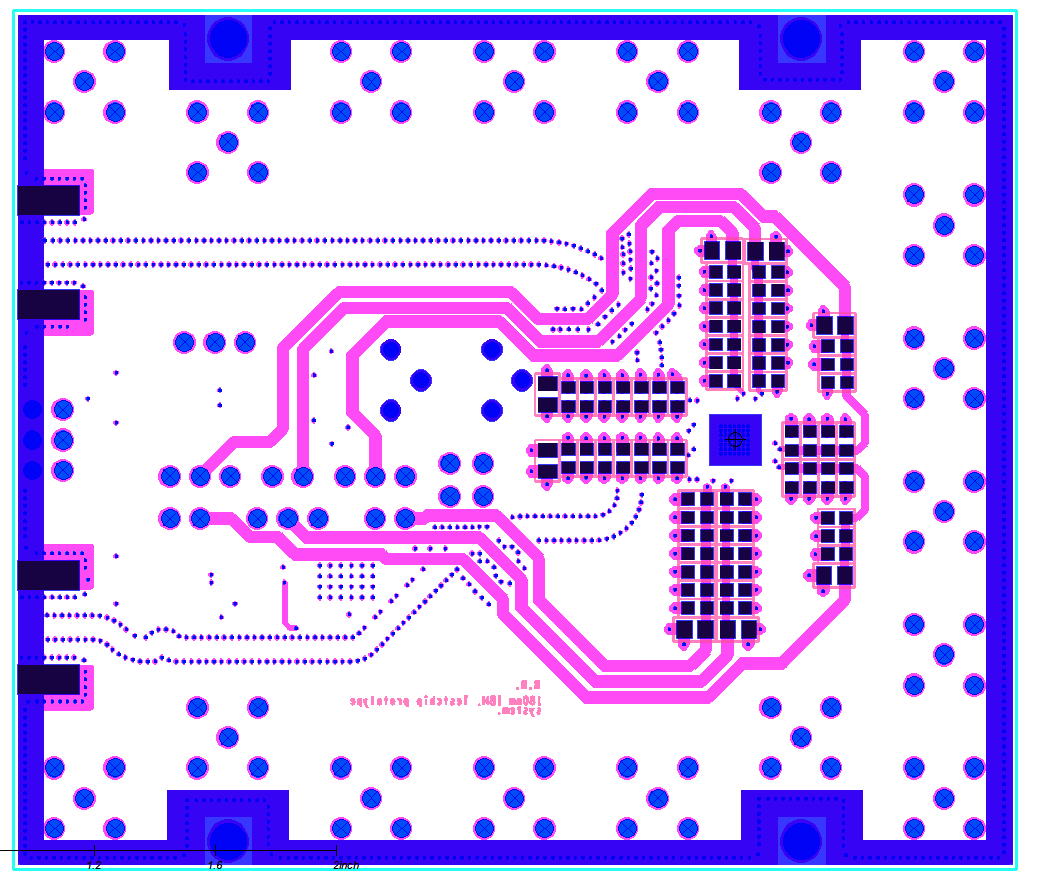Finally, here is the manufactured and assembled test PCB with the Digital Test Chip received from MOSIS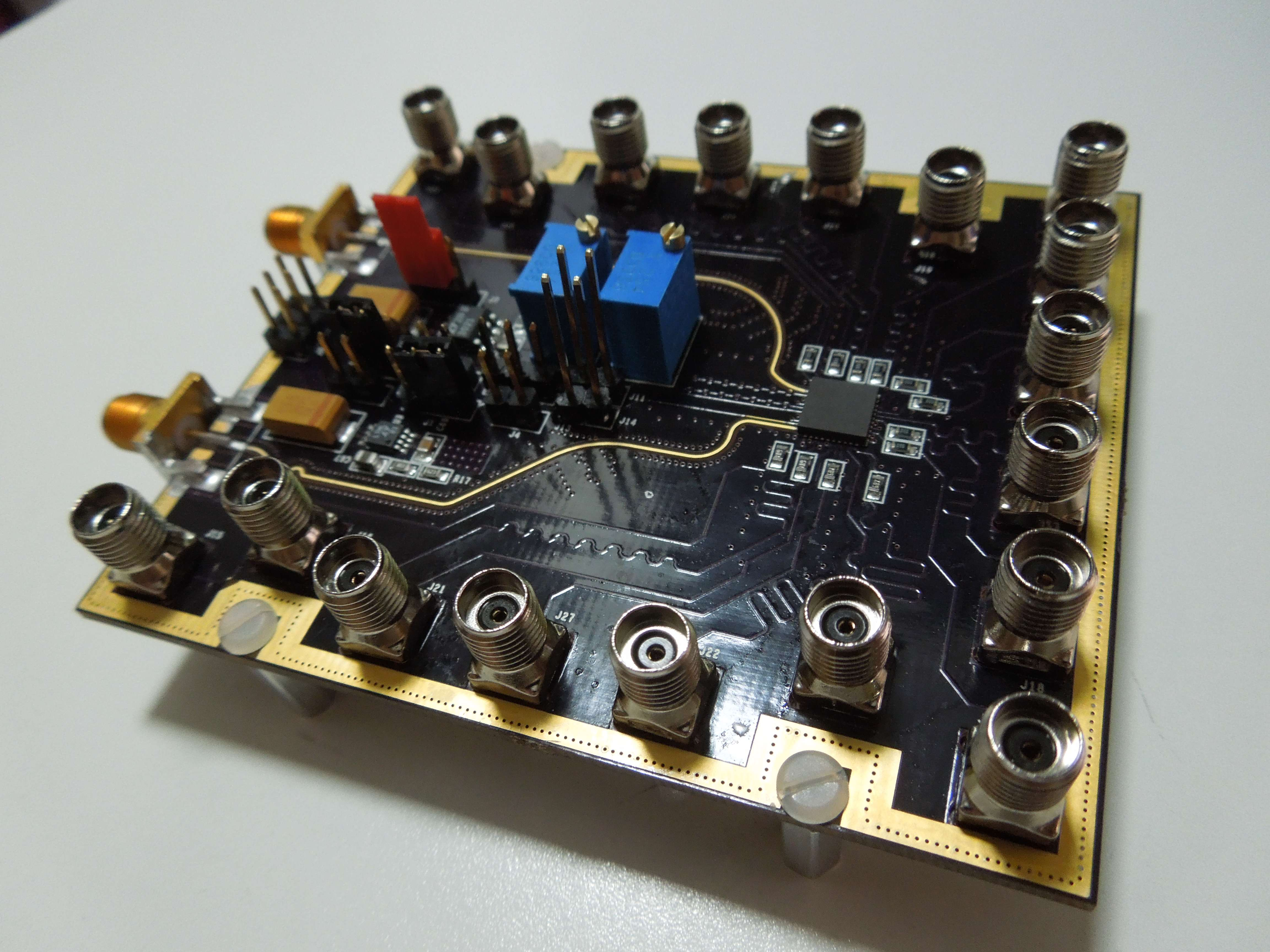Alright, it looks hot! Now it's time to play! Click Here for Experimental Results